Class 12 RD Sharma Solutions – Chapter 30 Linear Programming – Exercise 30.2 | Set 1

• Last Updated : 20 May, 2021

Question 1. Maximize Z = 5x + 2y,

Subject to

3x + 5y ≤ 15

5x + 2y ≤ 10

x, y ≥ 0

Solution:

Convert the given in equations into equations,

We will get the following equations:

3x + 5y = 15,

5x + 2y = 10,

x = 0 and

y = 0

Area represented by 3x + 5y ≤ 15:

The line 3x + 5y = 15 connects the coordinate axes at A(5,0) and B(0,3) respectively.

By connecting these points we will get the line 3x + 5y = 15.

Thus,

(0,0) assure the in equation 3x + 5y ≤ 15.

Hence,

The area having the origin shows the solution set of the in equation 3x + 5y ≤ 15.

Area shows by 5x + 2y ≤ 10:

The line 5x + 2y = 10 connects the coordinate axes at C(2,0) and D(0,5) respectively.

By connecting these points we will get the line 5x + 2y = 10.

Thus,

(0,0) satisfies the in equation 5x + 2y ≤ 10.

Hence,

The area having the origin shows the solution set of the in equation 5x + 2y ≤ 10.

Area shows by x ≥ 0 and y ≥ 0:

Here,

All the point in the first quadrant assures these in equations.

Thus,

The first quadrant is the area shows by the in equations x ≥ 0, and y ≥ 0.

The feasible area determined by the system of constraints, 3x + 5y ≤ 15, 5x + 2y ≤ 10, x ≥ 0, and y ≥ 0, are as follows.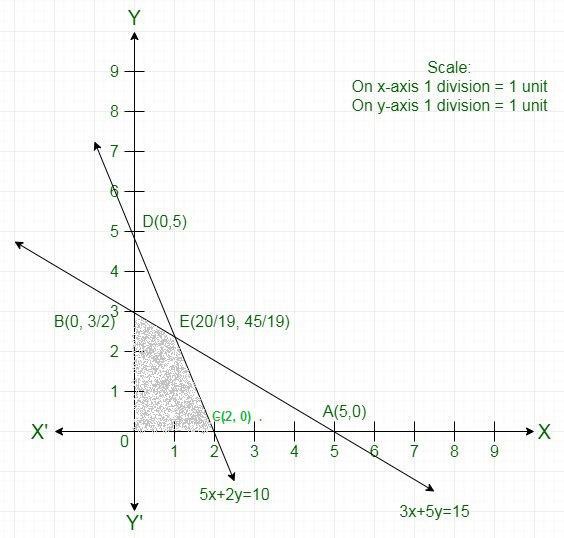The corner points of the feasible area are O(0, 0), C(2, 0), Eand B(0, 3).

The values of Z at these corner points are as follows.

Hence,

The maximum value of Zat the pointHence,

x=and y =is the best solution of the given LPP.

Therefore,

The best value of Z is.

Question 2. Maximize Z = 9x + 3y

Subject to

2x + 3y ≤ 13

3x + y ≤ 5

x, y ≥ 0

Solution:

Convert the given in equations into equations,

We will get the following equations:

2x + 3y = 13,

3x +y = 5,

x = 0 and

y = 0

Area shown by 2x + 3y ≤ 13:

The line 2x + 3y = 13 connects the coordinate axes at Aand Brespectively.

By connecting these points we will get the line 2x + 3y = 13.

Thus,

(0,0) assures the line equation 2x + 3y ≤ 13.

Thus, the area showing the origin represents the solution set of the in equation 2x + 3y ≤ 13.

The area shows by 3x + y ≤ 5:

The line 5x + 2y = 10 connects the coordinate axes at Cand D(0, 5) respectively.

After connecting these points,

We will get the line 3x + y = 5.

Thus,

(0,0) assures the in equation 3x + y ≤ 5.

Thus,

The area having the origin represents the solution set of the in equation 3x + y ≤ 5.

Area shown by x ≥ 0 and y ≥ 0:

Hence,

All the point in the first quadrant assures these in equations.

Thus,

The first quadrant is the area shown by the in equations x ≥ 0, and y ≥ 0.

The suitable area determined by the system of constraints,

2x + 3y ≤ 13,

3x + y ≤ 5,

x ≥ 0, and

y ≥ 0, are as follows.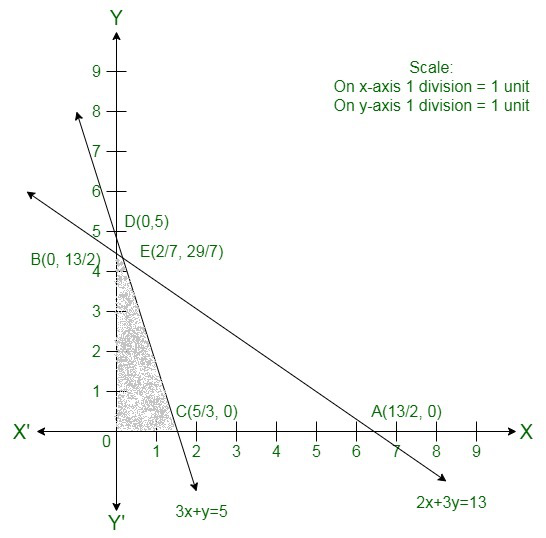The corner points of the suitable area are O(0, 0), C, Eand B.

The values of Z at these corner points are as follows.

Here,

We can see that the maximum value of the objective function Z is 15 which is at Cand E.

Thus,

The best value of Z is 15.

Question 3. Minimize Z = 18x + 10y

Subject to

4x + y ≥ 20

2x + 3y ≥ 30

x, y ≥ 0

Solution:

Convert the given in equations into equations,

We will get the following equations:

4x + y = 20,

2x +3y = 30,

x = 0 and

y = 0

Area shown by 4x + y ≥ 20 :

The line 4x + y = 20 connects the coordinate axes at A(5, 0) and B(0, 20) respectively.

By connecting these points we will get the line 4x + y = 20.

Thus,

(0,0) does not assure the in equation 4x + y ≥ 20.

Thus,

The area in xy plane which does not have the origin represents the solution set of the in equation 4x + y ≥ 20.

Area shown by 2x +3y ≥ 30:

The line 2x +3y = 30 connects the coordinate axes at C(15,0) and D(0, 10) respectively.

By joining these points we will get the line 2x +3y = 30.

Thus, (0,0) does not assure the in equation 2x +3y ≥ 30.

Thus,

The area which does not have the origin shows the solution set of the in equation 2x +3y ≥ 30.

Area shown by x ≥ 0 and y ≥ 0:

Hence,

Every point in the first quadrant assure these in equations.

Thus,

The first quadrant is the area shown by the in equations x ≥ 0, and y ≥ 0.

The suitable area determined by the system of constraints, 4x + y ≥ 20, 2x +3y ≥ 30, x ≥ 0, and y ≥ 0, are as follows.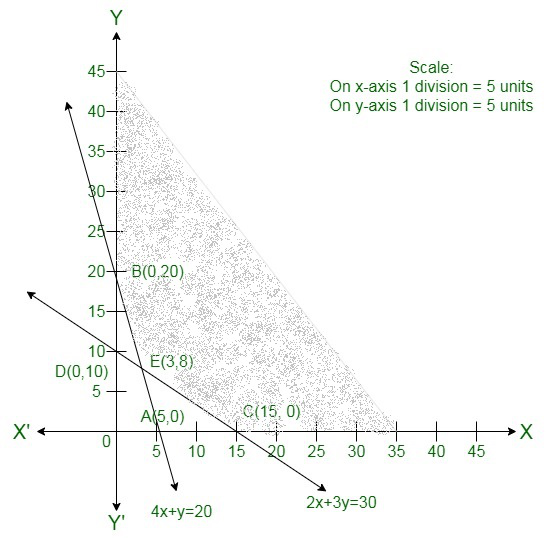The corner points of the suitable area are

B(0, 20),

C(15,0),

E(3,8) and

C(15,0).

The values of Z at these corner points are as follows.

Hence,

The minimum value of Z is 134 at the point E(3,8). Hence, x = 3 and y =8 is the best solution of the given LPP.

Therefore,

The best value of Z is 134.

Question 4.  Maximize Z = 50x + 30y

Subject to

2x + y ≤ 18

3x + 2y ≤ 34

x, y ≥ 0

Solution:

Convert the given in equations into equations,

We will get the following equations:

2x + y = 18, 3x + 2y = 34

The area shown by 2x + y ≥ 18:

The line 2x + y = 18 connects the coordinate axes at A(9, 0) and B(0, 18) respectively.

After connecting these points

We will get the line 2x + y = 18.

Thus,

(0,0) does not assure the in equation 2x + y ≥ 18.

Thus,

The region in xy plane which does not have the origin represents the solution set of the in equation 2x + y ≥ 18.

The area shown by 3x + 2y ≤ 34:

The line 3x + 2y = 34 connects the coordinate axes at C, 0 and D(0, 17) respectively.

After joining these points we will get the line 3x + 2y = 34.

Thus,

(0,0) assure the in equation 3x + 2y ≤ 34.

Thus,

The area having the origin shows the solution set of the in equation 3x + 2y ≤ 34.

The corner points of the suitable area are

A(9, 0), Cand E(2, 14).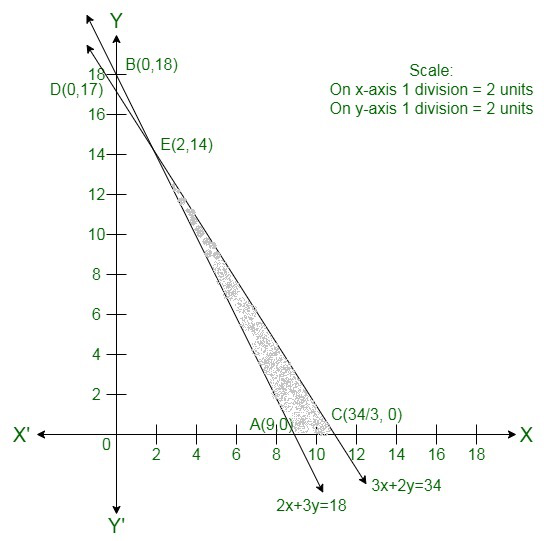The values of Z at these corner points are as follows.

Hence,

The maximum value of Z isat the point.

Hence,

x =and y = 0 is the best solution of the given LPP.

Therefore,

The best value of Z isQuestion 5. Maximize Z = 4x + 3y

Subject to

3x + 4y ≤ 24

8x + 6y ≤ 48

x ≤ 5

y ≤ 6

x, y ≥ 0

Solution:

Here we have to maximize Z = 4x + 3y

Convert the given in equations into equations,

We will get the following equations:

3x + 4y = 24,

8x + 6y = 48,

x = 5,

y = 6,

x = 0 and

y = 0.

The line 3x + 4y = 24 connects the coordinate axis at A(8, 0) and B(0,6).

Connect these points to get the line 3x + 4y = 24.

Thus,

(0, 0) assure the in equation 3x + 4y ≤ 24.

Thus,

The area in xy-plane that have the origin showing the solution set of the given equation.

The line 8x + 6y = 48 connects the coordinate axis at C(6, 0) and D(0,8). Connect these points to get the line 8x + 6y = 48.

Thus,

(0, 0) assures the in equation 8x + 6y  ≤ 48.

Thus,

The area in xy-plane that have the origin shows the solution set of the given equation.

x = 5 is the line passing through x = 5 parallel to the Y axis.

y = 6 is the line passing through y = 6 parallel to the X axis.

The area shown by x ≥ 0 and y ≥ 0:

Hence,

All the point in the first quadrant assure these in equations.

Thus,

The first quadrant is the area shows by the in equations.

These lines are drawn using a suitable scale.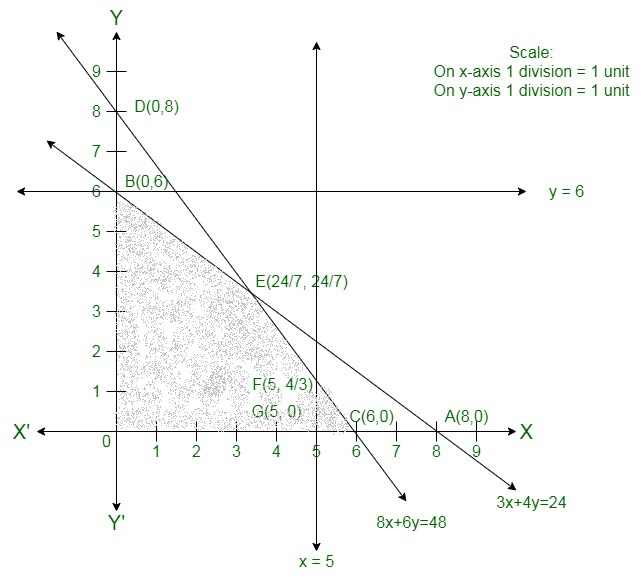The corner points of the suitable area are O(0, 0), G(5, 0), F, Eand B(0, 6).

The values of Z at these corner points are as follows.

Here we can see that the maximum value of the objective function Z is 24

Which is at Fand E

Therefore,

The best value of Z is 24.

Question 6. Maximize Z = 15x + 10y

Subject to

3x + 2y ≤ 80

2x + 3y ≤ 70

x, y ≥ 0

Solution:

Convert the given in equations into equations,

We will get the following equations:

3x + 2y = 80, 2x + 3y = 70, x = 0 and y = 0

The area shown by 3x + 2y ≤ 80 :

The line 3x + 2y = 80 connects the coordinate axes at A, 0 and B(0, 40) respectively.

By connecting these points we will get the line 3x + 2y = 80.

Thus,

(0,0) assure the in equation 3x + 2y ≤ 80 .

Thus,

The area having the origin represents the solution set of the in equation 3x + 2y ≤ 80 .

The area shown by 2x + 3y ≤ 70:

The line 2x + 3y = 70 connects the coordinate axes at C(35, 0)C35, 0 and D(0, 703)D0, 703 respectively.

After connecting these points we will get the line 2x + 3y ≤ 70.

Thus,

(0,0) assure the in equation 2x + 3y ≤ 70.

Thus,

The area having the origin shows the solution set of the in equation 2x + 3y ≤ 70.

The area shown by x ≥ 0 and y ≥ 0:

Hence,

Every point in the first quadrant assure these in equations.

Thus,

The first quadrant is the area shown by the in equations x ≥ 0 and y ≥ 0.

The suitable area determined by the system of constraints, 3x + 2y ≤ 80, 2x + 3y ≤ 70, x ≥ 0, and y ≥ 0 are as follows.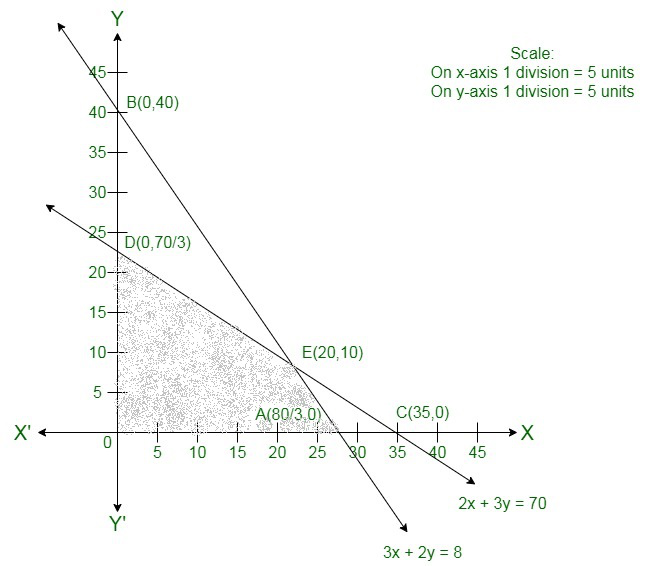The corner points of the suitable area are O(0, 0), A, 0 ,E(20, 10) and D.

The values of Z at these corner points are as follows.

Thus we can see that the maximum value of the objective function Z is 400 which is at Aand E(20, 10).

Therefore,

The best value of Z is 400.

Question 7.  Maximize Z = 10x + 6y

Subject to

3x + y ≤ 12

2x + 5y ≤ 34

x, y ≥ 0

Solution:

Convert the given in equations into equations,

We will get the following equations:

3x + y = 12, 2x + 5y = 34, x = 0 and y = 0

The area shown by 3x + y ≤ 12:

The line 3x + y = 12 connects the coordinate axes at A(4, 0) and B(0, 12) respectively.

After connecting these points we will get the line 3x + y = 12.

Thus,

(0,0) assure the in equation 3x + y ≤ 12.

Thus,

The area having the origin represents the solution set of the in equation 3x + y ≤ 12.

The area shown by 2x + 5y  ≤ 34:

The line 2x + 5y = 34 connects the coordinate axes at C(17, 0) and Drespectively.

After connecting these points we will get the line 2x + 5y ≤ 34.

Thus,

(0,0) assure the in equation 2x + 5y ≤ 34.

Thus,

The area having the origin represents the solution set of the in equation 2x + 5y ≤ 34.

Area having by x ≥ 0 and y ≥ 0:

Hence,

All the point in the first quadrant assure these in equations.

Thus,

The first quadrant is the area shown by the in equations x ≥ 0 and y ≥ 0.

The suitable area determined by the system of constraints, 3x + y ≤ 12, 2x + 5y ≤ 34, x ≥ 0, and y ≥ 0 are as follows.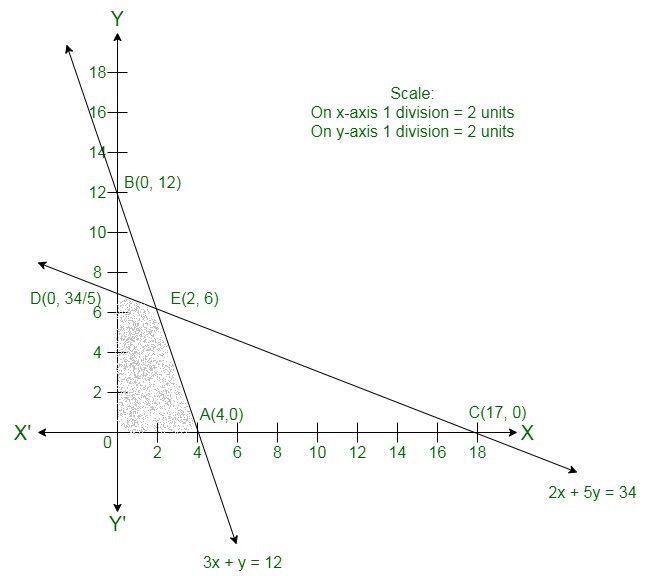The corner points of the suitable area are O(0, 0), A(4, 0) ,E(2, 6) and D.

The values of Z at these corner points are as follows:

Here we can see that the maximum value of the objective function Z is 56 which is at E(2, 6) that means at x = 2 and y = 6.

Therefore,

The best value of Z is 56.

Question 8.  Maximize Z = 3x + 4y

Subject to

2x + 2y ≤ 80

2x + 4y ≤ 120

Solution:

Here we have to maximize Z = 3x + 4y

Convert the given in equations into equations, we will get the following equations:

2x + 2y = 80, 2x + 4y = 120

The area shown by 2x + 2y ≤ 80:

The line 2x + 2y = 80 connects the coordinate axes at A(40, 0) and B(0, 40) respectively.

After connecting these points we will get the line 2x + 2y = 80.

Thus,

(0,0) assure the in equation 2x + 2y ≤ 80.

Thus,

The area having the origin represents the solution set of the in equation 2x + 2y ≤ 80.

The area shown by 2x + 4y ≤ 120:

The line 2x + 4y = 120 connects the coordinate axes at C(60, 0) and D(0, 30) respectively.

After connecting these points we will get the line 2x + 4y ≤ 120.

Thus,

(0,0) assure the in equation 2x + 4y ≤ 120.

Thus,

The area having the origin represents the solution set of the in equation 2x + 4y ≤ 120.

The suitable area determined by the system of constraints, 2x + 2y ≤ 80, 2x + 4y ≤ 120 are as follows: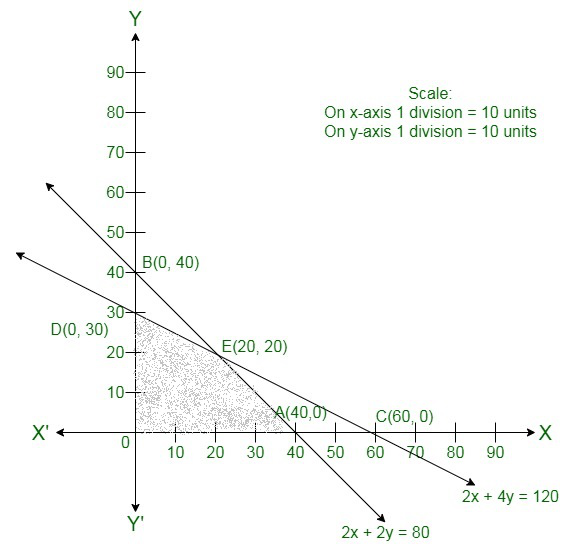The corner points of the suitable area are O(0, 0), A(40, 0), E(20, 20) and D(0, 30).

The values of Z at these corner points are as follows:

Here we can see that the maximum value of the objective function Z is 140 which is at E(20, 20) that means at

x = 20 and y = 20.

Therefore,

The best value of Z is 140.

Question 9. Maximize Z = 7x + 10y

Subject to

x + y ≤ 30000

y ≤ 12000

x ≥ 6000

x ≥ y

x, y ≥ 0

Solution:

Here we have to maximize Z = 7x + 10y

Convert the given in equations into equations,

We will get the following equations:

x + y = 30000,y = 12000, x = 6000, x = y, x = 0 and y = 0.

Region represented by x + y ≤ 30000:

The line x + y = 30000 connects the coordinate axes at A(30000, 0) and B(0, 30000) respectively.

After connecting these points we will get the line x + y = 30000.

Thus, (0,0) assure the in equation x + y ≤ 30000.

Thus,

the area having the origin represents the solution set of the in equation x + y ≤ 30000.

The line y = 12000 is the line that passes through C(0,12000) and parallel to x axis.

The line x = 6000 is the line that passes through (6000, 0) and parallel to y axis.

The area shown by x ≥ y

The line x = y is the line that passes through origin.The points to the right of the line x = y assure the inequation x ≥ y.

Like by taking the point (−12000, 6000).

Here, 6000 > −12000 which implies y > x.

Hence,

the points to the left of the line x = y will not assure the given inequation x ≥ y.

The area shown by x ≥ 0 and y ≥ 0:

Hence,

All the point in the first quadrant assure these inequations.

Thus,

the first quadrant is the area shown by the inequations x ≥ 0 and y ≥ 0.

The suitable area determined by the system of constraints, x + y ≤ 30000, y ≤ 12000, x ≥ 6000, x ≥  y , x ≥ 0 and y ≥ 0 are as follows: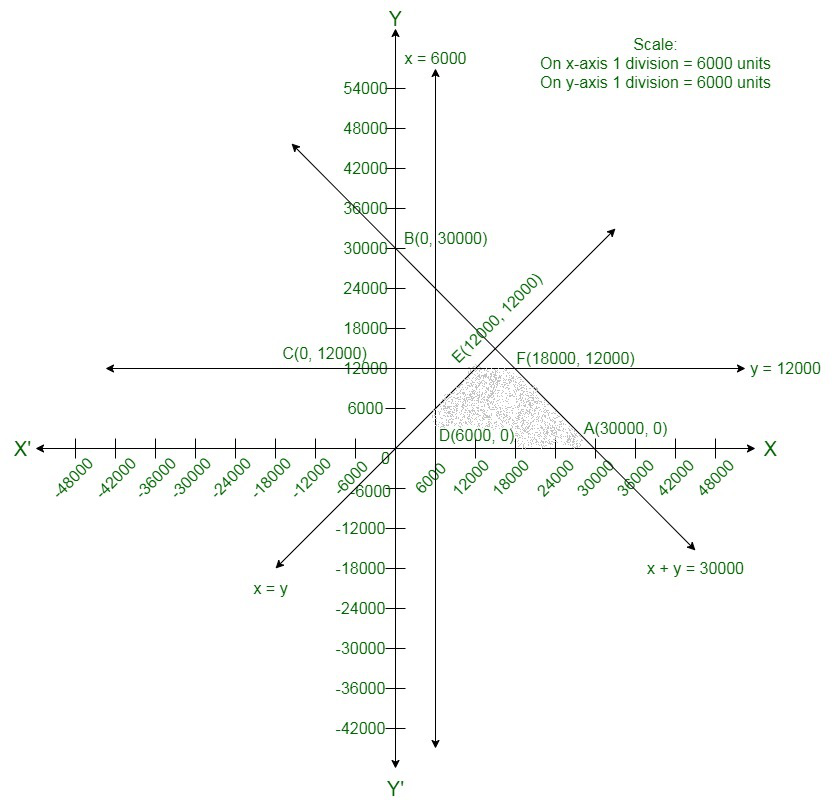The corner points of the feasible region are

D(6000, 0),

A(3000,

F(18000, 12000) and

E(12000, 12000).

The values of Z at these corner points are as follows:

Here we can see that the maximum value of the objective function Z is 246000 which is at F(18000, 12000) that means at

x = 18000 and y = 12000.

Therefore,

The best value of Z is 246000.

Question 10. Minimize Z = 2x + 4y

Subject to

x + y ≥ 8

x + 4y ≥ 12

x ≥ 3, y ≥ 2

Solution:

Convert the given in equations into equations, we will get the following equations:

x + y = 8, x + 4y = 12, x = 3, y = 2

The area shows by x + y ≥ 8:

The line x + y = 8 connects the coordinate axes at A(8, 0) and B(0, 8) respectively.

After connecting these points we will get the line x + y = 8.

Thus,

(0,0) does not assure the in equation x + y ≥ 8.

Thus,

The region in xy plane which does not contain the origin represents the solution set of the in equation x + y ≥ 8.

The area shown by x + 4y ≥ 12:

The line x + 4y = 12 connects the coordinate axes at C(12, 0) and D(0, 3) respectively.

After connecting these points we will get the line x + 4y = 12.

Thus,

(0,0) assure the in equation x + 4y ≥ 12.

Thus,

the area in xy plane which having the origin represents the solution set of the in equation x + 4y ≥ 12.

The line x = 3 is the line that passes through the point (3, 0) and is parallel to Y axis.x ≥ 3 is the area to the right of the line

x = 3.

The line y = 2 is the line that passes through the point (0, 12) and is parallel to X axis.y ≥ 2 is the area above the line y = 2.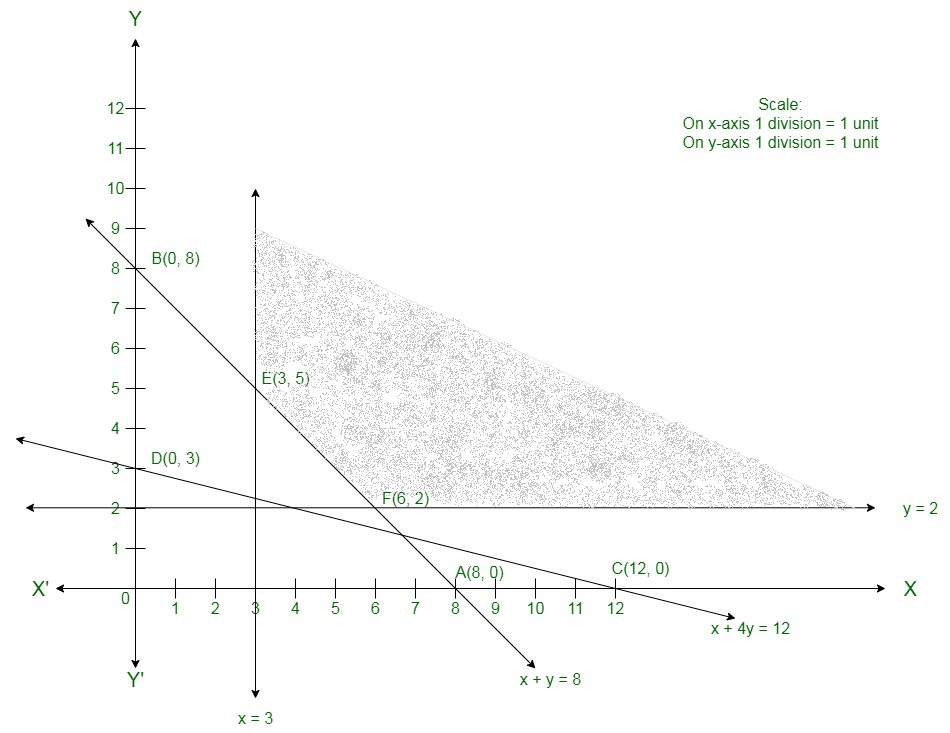The corner points of the suitable region are E(3, 5) and F(6, 2).

The values of Z at these corner points are as follows.

Therefore,

The minimum value of Z is 20 at the point F(6, 2).

Hence, x = 6 and y =2 is the best solution of the given LPP.

Therefore,

The best value of Z is 20.

My Personal Notes arrow_drop_up